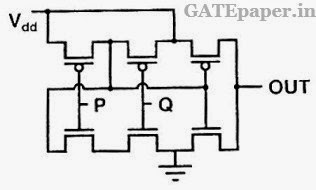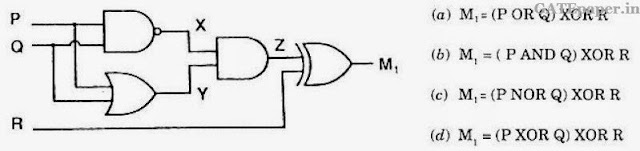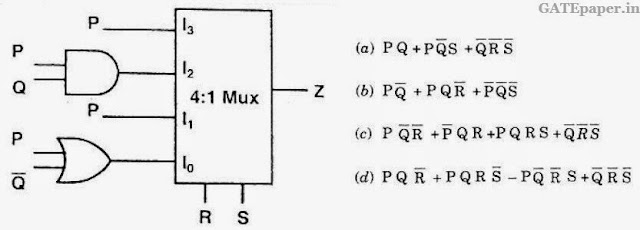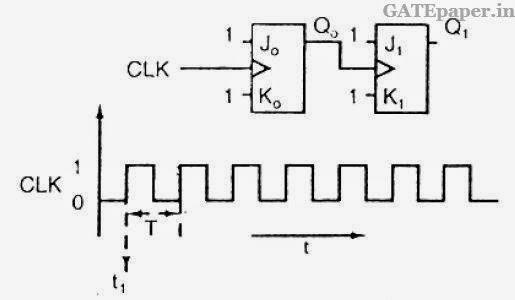### GATE 2008 ECE Video Solution on Digital Circuits (Digital Electronics)

1. An 8085 executes the following instructions
2710 LXI H, 30A0H
2714 PCHL
All addresses and constants are in Hexa decimal. Let PC be the contents of the program counter and HL be the contents of HL register pair just after executing PCHL.
Which of the following statements is correct?
a. PC = 2715H and HL = 30A0H
b. PC = 30A0H and HL = 2715H
c. PC = 6140H and HL = 6140H
d. PC = 6140H and HL = 2715H

2. The logic function implemented by the following circuit at the terminal OUT isa. P NOR Q
b. P NAND Q
c. P OR Q
d. P AND Q

3. The two numbers represented in signed 2’s compliment form are P = 11101101 and Q = 11100110. If Q is subtracted from P, the value obtained in signed 2’s compliment form is
a. 100000111
b. 00000111
c. 11111001
d. 111111001

4. Which of the following Boolean expression correctly represents the relation between P, Q, R and M1?5. For the circuit shown in the following figure, I0 – I3 are inputs to the 4:1 multiplexer, R(MSB) and S are control inputs. The output Z can be represented by6. For each of the positive edge triggered JK flip flop used in the following figure, the propagation delay is ΔT.Which of the following waveforms correctly represents the output at Q1?7. For the circuit shown in the figure, D has a transition from 0 to 1 after CLK changes from 1 to 0. Assume gate delays to be negligible.Which of the following statements is TRUE?
a. Q goes to 1 at the CLK transition and stays at 1
b. Q goes to 0 at the CLK transition and stays at 0
c. Q goes to 1 at the CLK transition and goes to 0 when D goes to 1
d. Q goes to 0 at the CLK transition and goes to 1 when D goes to 1

In the following circuit, the comparator output is logic ‘1’ if V+ > V- and is logic ‘0’
otherwise. The D/A conversion is done as per the relation.The counter starts from the clear state.8. The stable reading of the LED display is
a. 06
b. 07
c. 12
d. 13
9. The magnitude of the error between VDAC and Vin at steady state in volts is
a. 0.2
b. 0.3
c. 0.5
d. 1.0
Solution (8 & 9) : https://www.youtube.com/watch?v=8WEFmNeqxYI